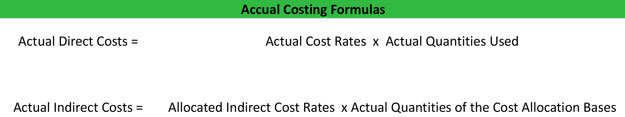# What is Actual Costing?

Definition: Actual costing is a cost accounting system that uses actual cost, direct-cost rates, and actual qualities used in production to determine the cost of specific products. Usually an actual costing system traces direct costs to a cost object or something that has a measurable cost.

In other words, managers go back to the source of the costs (cost objects) like labor and materials. Managers can analyze how many hours of manufacturing time a product requires to calculate the actual costs of producing that product.

## What Does Actual Costing Mean?

Here are the typical actual costing system formulas:## Example

Managers can use these formulas to calculate the total production costs. For instance, managers first need to find out how many hours it took the company to produce the product and how much the company is paying its employees per hour. Using the first actual costing formula, these numbers make up the labor portion of the production costs. The same is done for materials. Overhead is a little different.

Since overhead like utility usage is a little difficult to assign to a single product, managers usually make estimates. They estimate how much overhead was used and how long the overhead was used. Using the second actual costing formula, management can determine the indirect productions costs for producing the product. After all the calculations are done, add up the totals and you’ll get the actual cost of producing your product.

After the actual cost is known, management can change the production process in order to meet budget goals.